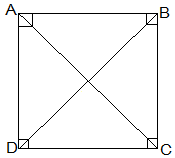Guru

# ABCD is a rectangle in which diagonal AC bisects ∠A as well as ∠C. Show that: (i) ABCD is a square Q.8(1)

• 0

Yesterday i was doing the question from class 9th ncert book of math of Quadrilaterals chapter of exercise 8.1  What is the easiest way for solving it because i was not able to do this question please help me for solving this question ABCD is a rectangle in which diagonal AC bisects ∠A as well as ∠C. Show that: (i) ABCD is a square

Share

1.(i) ∠DAC = ∠DCA (AC bisects ∠A as well as ∠C)

⇒ AD = CD (Sides opposite to equal angles of a triangle are equal)

also, CD = AB (Opposite sides of a rectangle)

,AB = BC = CD = AD

Thus, ABCD is a square.

• 0